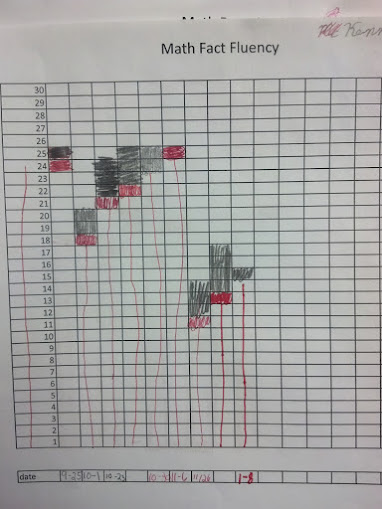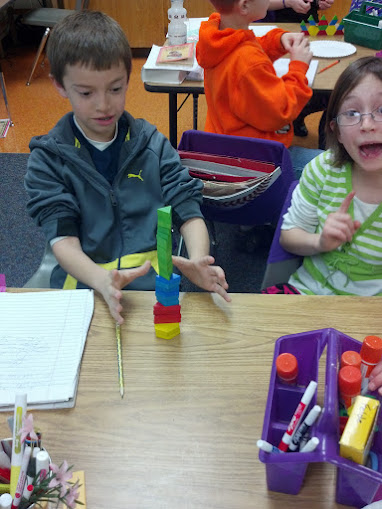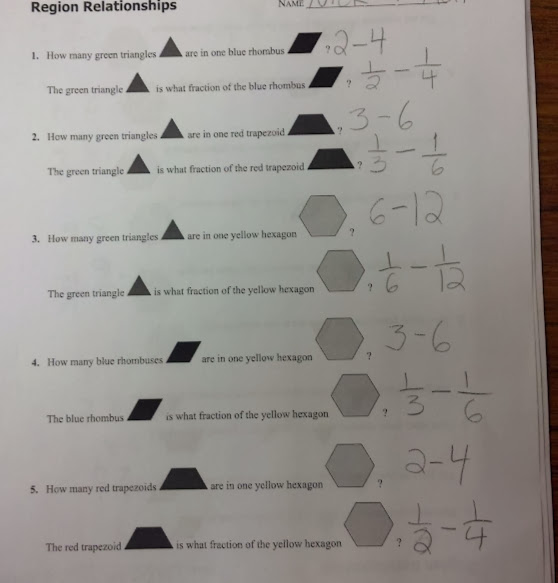# Pattern Blocks to Investigate Fractions

23 teachers like this lesson
Print Lesson

## Objective

SWBAT identify fractions when the whole (region) and a part of the region are given and understand numerator and denominator.

#### Big Idea

In this hands on lesson, students explore fractional relationships using pattern blocks in order to build conceptual understanding of numerator and denominator.

## Number Hook

5 minutes

The most important thing to be learned from my number hooks and magic tricks is that students will pick up and explore material that interests them.  Magic is a fabulous and fun way of making math come alive for my students. It allows for a true inquiry environment for learning.

I start this warm up using this number trick with multiples of 9.

My students have seen a very similar trick to this before.  They love it. So far, none of my students have figured out exactly how it works, but some are getting very close.

The magic of nines is captivating for students and adults. For more information about nines, you can look up "casting out nines" for other fun math magic tricks.  This one is quite simple. For any number, when you add the digits together and then subtract this number from the original number, the result will be a multiple of nine. The gopher trick uses this principal.  Every multiple of nine has the same symbol.  Students don't notice this because they are concentrating so much on their number.  The gopher then guesses the symbol correctly every single time.

## Warm Up

7 minutes

Students will start today's lesson with a fluency assessment.  This assessment is from Monitoring Basic Skills Progress Second Edition: Basic Math Computation by Lynn S. Fuchs, Carol L. Hamlett, and Douglas Fuchs.

This is an assessment I have my students do each week and then graph their results.  It allows them to reflect on their learning of basic math facts, as well as using all four operations with whole numbers, and adding and subtracting unit fractions.  (It also happens to be the quietest time in my math classroom all week!!)

This is what my classroom looks like as students work on this assessment.

This is sample of a student's graph. You can also click here to hear my thoughts about this graph below.Click here to see an example of a typical fourth grade fluency decrease since students are completing the fourth grade fluency set that incorporates division, multiplication, addition, subtraction, and adding and subtracting fractions with like denominators. (At this point, students have not had many fraction lessons, thus very very students are able to complete the fraction problems)

I do not start my students with the fourth grade skills, but at this point in the year, all students are using the fourth grade set.. I chose to start them with the end of the third grade skills which covers addition, subtraction and multiplication and division of basic facts. I strongly believe in a balanced math approach, which is one reason why I also believe in common core standards.  By having a balance of building conceptual understanding, application of problems, and computational fluency, students can experience rigorous mathematics.  I want to make clear that this assessment ONLY measures basic math computation.  It is only one piece of students' knowledge. The assessments in this book, for each grade level, do not change in difficulty over the course of the year.  Therefore, a student's increase in score over the school year truly reflects improvement in the student's ability to work the math problems at that grade level.

## Concept Development

45 minutes

This lesson is adapted from the illuminations website. My goal for this lesson is that students are able to express in their own words the definition of a numerator and denominator after building fractions with pattern blocks.

I begin this lesson by passing a set of pattern blocks to partners.  (Only the yellow hexagons, red trapezoids, blue rhombi, and green triangles are needed. Students do not use the orange triangle or the tan rhombus for this lesson.) I give them about 4 hexagons, 18 triangles, 6 rhombi, and 6 trapezoids. I then give them about 5 minutes to "play" with the blocks. I have found from past experience that students needs a few minutes to explore the manipulatives, build with them, touch each one, and basically play and manipulate them on their own terms before they are ready to use the blocks as a learning tool.

You can see here how these two are really enjoying their "play" time.After five minutes, students work in pairs to explore fraction relationships. Students use pattern blocks to find relationships and to determine the answer using sheet FunFractions-AS-RegionRelationships2.

The worksheet guides students by asking questions about the fractional relationship.

For example:

How many green trianglesare in one blue rhombus? [Two.]

The green triangleis what fraction of the blue rhombus? [One out of two, or ½.]

I then model the written form of each fraction by recording each fraction on the board. For example,

 2= 1Therefore, 1= ½My students should have little difficulty expressing this relationship as a fraction since they have used the fraction ½ on many occasions even prior to kindergarten. This lesson focuses on the written format and what the numerator and denominator really mean, building students conceptual understanding of fractions.

You can see a sample of students' work below. The second column of numbers were from an extension I asked groups to do if they finished early. I asked groups to change the size of the "whole" to two of the shapes, instead of one.  It appears that the student is subtracting, but he/she is simply noting that if there were two of each whole, like two rhombi, and still one triangle, the triangle would then represent 1/4 of the whole.When most students are finished I lead a whole class discussion asking questions like the ones found in this resource - fraction questions pattern blocks.  During the discussion I write the fraction as students list the number of parts in the whole.  this helps students understanding of where to place the numerator and denominator when writing fractions in standard form.

## Student Debrief

7 minutes

For this wrap up I give my students an exit ticket .  On the exit ticket, I instruct my students to describe in their own words what a numerator means and what does a denominator mean?

From this exit ticket, I will make small groups for re-teaching.

Note:  When analyzing the exit tickets, I sorted them into two piles - students that understand both terms and students who do not.  After sorting the exit tickets, I had 8 students in the pile of students who did not understand both terms. I think some of those students are close, but not yet where I want them to be in their explanation or communication about these words.  This is crucial for students to understand.  Many math problems and concepts in the future rely on students understanding numerator and denominator.  For example, much later in the spring, students will find 1/6 of 180 degrees when we work with angles - thy must have a solid understanding of denominator in order to be successful.